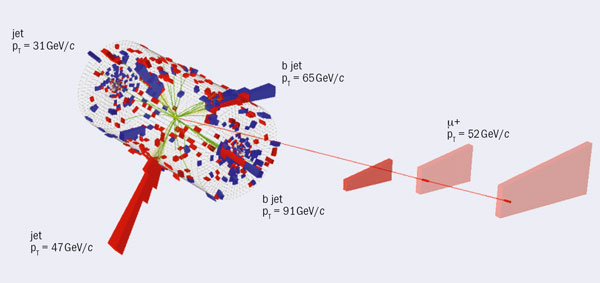# Looking at the top for new physics

23 February 2012

Last year one of the properties of top-quark production, the tt charge asymmetry, attracted much interest with the publication of measurements by the CDF and DØ collaborations at Fermilab’s Tevatron (T Aaltonen et al. 2011, V M Abazov et al. 2011). They reported results that were 2σ above the predicted values. This deviation can be explained by several theories that go beyond the Standard Model by introducing new particles that contribute to top-quark production. The CMS collaboration has now measured this top-quark property for the first time at the LHC – and finds a different result.Fig. 1. Event display of a tt̅ candidate event. The t is reconstructed in the forward direction; the t̅ is reconstructed from the three central jets. The difference in pseudorapidity Δ|η| is 2.7.

In the Standard Model, a difference in angular distributions between top quarks and antiquarks (commonly referred to as the charge asymmetry) in tt production through quark–antiquark annihilation appears in QCD calculations at next-to-leading order. It leads to there being more top quarks produced at small angles to the beam pipe, while top antiquarks are produced more centrally (figure 1). As a consequence, the pseudorapidity distribution of top quarks is broader than that of top antiquarks, which makes the difference of the respective pseudorapidities, Δ|η| = |ηt| – |ηt|, a suitable observable for measuring the charge asymmetry (figure 2). The Standard Model predicts a small asymmetry of AC = 0.0136 ± 0.0008, which translates into an excess of about 1% of events with Δ|η| > 0 compared with events with Δ|η| < 0 (Kuhn and Rodrigo 2011). The existence of new sources of physics could enhance this asymmetry.Fig. 2. The reconstructed distribution of the difference of the absolute values of the top quark and antiquark pseudorapidities.

CMS has measured the tt charge asymmetry using data corresponding to an integrated luminosity of 1 fb–1 (CMS collaboration 2011). A total of 12 757 events were selected in the lepton+jets channel, where one top quark decays into a b quark, a charged lepton (electron or muon) and the corresponding neutrino, while the other top quark decays into three quarks. The background contribution to this dataset is about 20%. The measurement of the charge asymmetry is based on the full reconstruction of the four-momenta of the top quarks, which have to be reconstructed from the observed leptons, jets and missing transverse energy. The dependency of the selection efficiency both on the Δ|η| value and on the smearing of the momenta of the top-quark decay products, because of finite detector resolution, are accounted for when calculating the final result.

The measured value of AC = –0.017 ± 0.032 (stat.)+0.025 –0.036 (syst.) is consistent with the Standard Model prediction and does not provide any indication for a new physics contribution. CMS also measured the uncorrected charge asymmetry as a function of the invariant mass of the top-quark pair, mtt. Previous measurements by the CDF collaboration had found an asymmetry that was more than 3σ above the predicted value for large values of mtt. However, the current analysis by CMS dampens the excitement that the CDF result caused because it reveals no hints for a deviation from the Standard Model predictions.